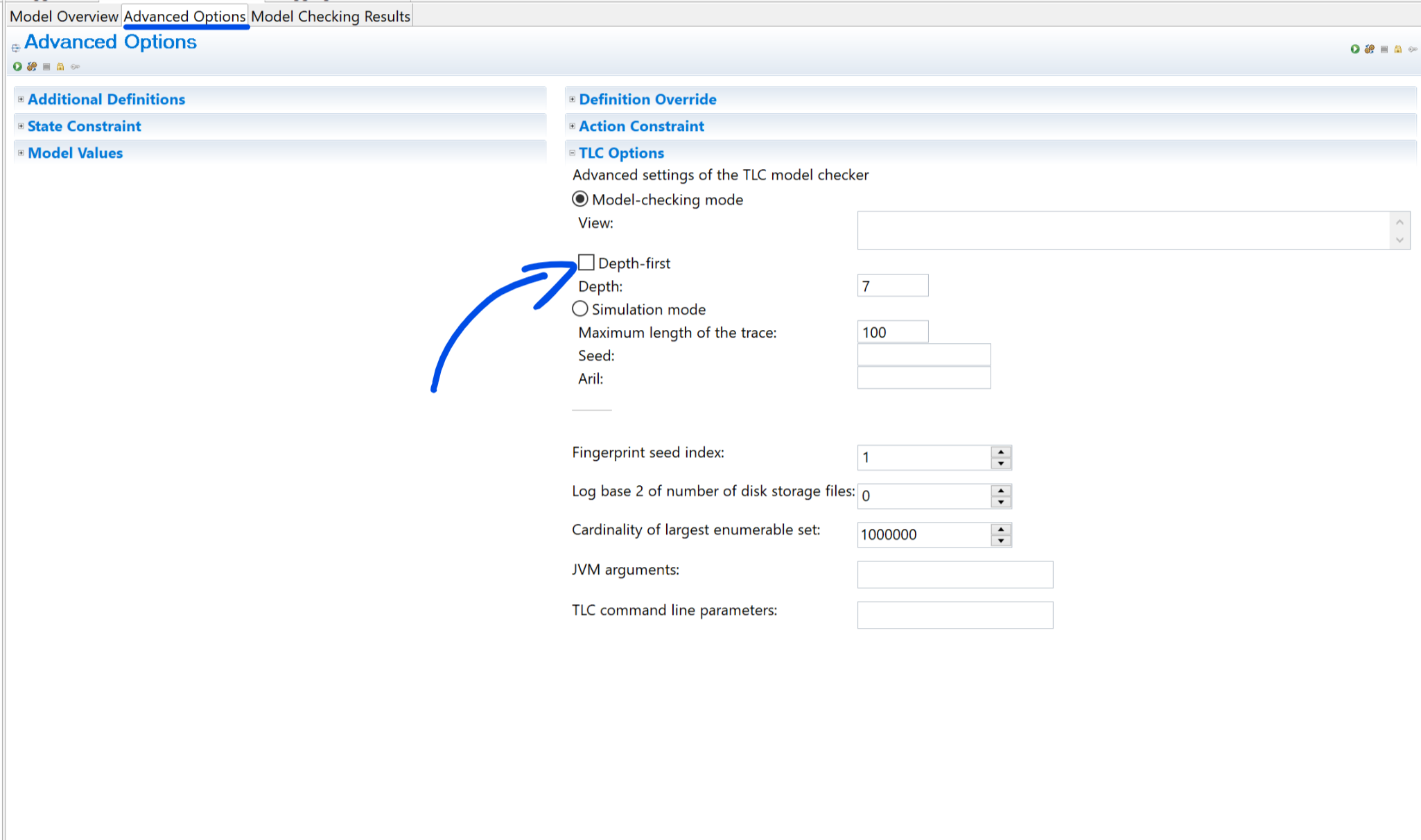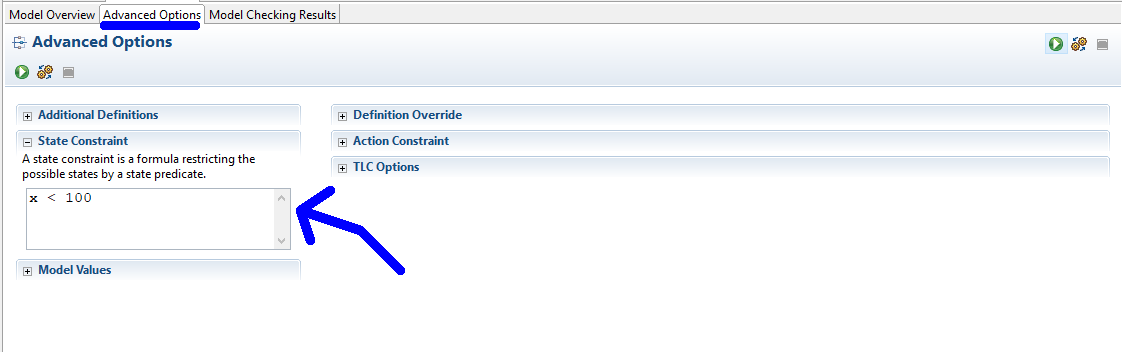# Infinite Loops

Infinite loops are useful to model, as they are a great way of representing things like cronjobs or worker pools. You can add infinite loops in the same way as any other language, by using `while` or `goto`.

``````process whileprocess = "while"
begin While:
while TRUE do
skip
end while
end process

process gotoprocess = "goto"
begin Goto:
skip;
goto Goto;
end process;
``````

There are two specific problems with using infinite loops in TLA+. The first is that if the infinite loop produces a state change, TLC might try to check an infinite state space. The second is that it makes checking termination harder: your program is done when everything but the infinite loop has terminated.

### State Space Restriction

If we try to check the following spec, TLC will run out of memory:

``````variable x = 0;
while TRUE do
x := x + 1;
end while
end algorithm;
``````

There’s an infinite number of possible states here: `x = 1`, `x = 2`, etc. The naive way of fixing this would be to add a time counter:

``````variable x = 0, time = 0;
However, this can quickly get untenable. Another options is to use the regular loops and switch to a depth-first search. This lets you force the model to terminate in a finite number of steps.The last option is to use a state constraint. A state constraint is a formula restricting the possible states: When a state does not comply with the constraint, model will finish running.